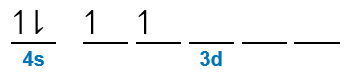# Problem: Give a possible set of values of the four quantum numbers for the 4s and 3d electrons in titanium.

###### FREE Expert Solution

Titanium Electron Configuration:

Ti (atomic # = 22):       [Ar]4s23d2principal quantum number (n)  energy level in orbitals and its value could be any positive integer starting from 1 to infinity

angular momentum quantum number (ℓ) → (l) has to be at least 1 less than n, range of values from 0 up to (n-1)

▪ Each ℓ value corresponds to a subshell:

= 0 → s subshell
= 1 → p subshell
= 2 → d subshell
= 3 → f subshell

• magnetic quantum number (m) → range of values from - to +

▪ The m values (for each ℓ) represent an orbital for the subshell:

s subshell→ 1 orbital
p subshell→ 3 orbitals
d subshell → 5 orbitals
f subshell → 7 orbitals

spin quantum number (ms) → can only have two possible values +½ (spin up electron) or ½ (spin down electrons)

• Note that 1 orbital can hold a maximum of 2 electrons → 1 spin up (+½) and 1 spin down (–½)

For the 2s electrons:

4s → n = 4

▪ s subshell →  = 0

• for mrange of values from - to +

▪ since s subshell has only one orbital:    m → has only one value
m = 0

1 spin up electron → ms = +½
1 spin down electron → ms = –½

Possible Quantum Numbers:

n = 4            ℓ = 0               m = 0            ms = +½
n = 4            ℓ
= 0               m = 0            ms = –½

For the 3d electrons:

85% (300 ratings)###### Problem Details

Give a possible set of values of the four quantum numbers for the 4s and 3d electrons in titanium.

Frequently Asked Questions

What scientific concept do you need to know in order to solve this problem?

Our tutors have indicated that to solve this problem you will need to apply the The Electron Configuration: Quantum Numbers concept. You can view video lessons to learn The Electron Configuration: Quantum Numbers. Or if you need more The Electron Configuration: Quantum Numbers practice, you can also practice The Electron Configuration: Quantum Numbers practice problems.

What professor is this problem relevant for?

Based on our data, we think this problem is relevant for Professor Awino's class at ISU.

What textbook is this problem found in?

Our data indicates that this problem or a close variation was asked in Chemistry: An Atoms First Approach - Zumdahl Atoms 1st 2nd Edition. You can also practice Chemistry: An Atoms First Approach - Zumdahl Atoms 1st 2nd Edition practice problems.